#### 期刊菜单

Design of Motion Profile Controller for Permanent Magnet Synchronous Linear Motor

Abstract: Aiming at the problem of nonlinear friction interference of permanent magnet synchronous linear motor (PMLSM) in motion, because the traditional PID control cannot meet the high control requirements, this paper proposes a profile controller based on the combination of sliding mode controller (SMC) and Disturbance observer (DOB), which can effectively suppress interference and improve the accuracy of motion profile. SMC is a variable structure control with strong robustness, while DOB is able to effectively suppress external interference. The mathematical model of permanent magnet synchronous linear motor is given, and after simulation and analysis on Simulink, it shows that the control system has good robustness and good motion profile.

1. 引言

2. 永磁同步直线电机数学建模

1) 直线电机所在温度变化对直线电机的工作影响忽略不计；

2) 直线电机上的三项绕组成对称分布，并且在空间上相差，忽律电机运行过程中而产生的磁动势；

3) 绕组的自感和互感始终保持不变；

4) 忽略直线电机的一些损耗，如磁滞，铁芯和涡流；

5) 直线电机的定子空载电势呈正弦波，忽略磁场高次谐波。

dq坐标系建立在永磁体上，d轴与永磁体的磁感应线重合，坐标随着永磁体的转动相对于动子旋转。其数学模型可以由三相静止坐标系下的数学模型通过Clark变换和旋转变换得来。

$\left\{\begin{array}{l}{u}_{d}={R}_{s}{i}_{d}+p{\psi }_{d}-{w}_{e}{\psi }_{q}\\ {u}_{q}={R}_{s}{i}_{q}+p{\psi }_{q}-{w}_{e}{\psi }_{d}\end{array}$ (1)

${w}_{e}=\frac{\pi }{\tau }{v}_{e}$ (2)

$\left\{\begin{array}{l}{\psi }_{d}={L}_{d}{i}_{d}+{\psi }_{f}\\ {\psi }_{q}={L}_{q}{i}_{q}\end{array}$ (3)

${F}_{e}=\frac{3}{2}\frac{\pi }{\tau }\left[{\psi }_{f}{i}_{q}+\left({L}_{d}-{L}_{q}\right){i}_{d}{i}_{q}\right]$ (4)

${F}_{e}=\frac{3}{2}\frac{\pi }{\tau }{\psi }_{f}{i}_{q}={k}_{F}{i}_{q}$ (5)

$M\frac{\text{d}v}{\text{d}t}+f+Bv={F}_{e}$ (6)

$\left\{\begin{array}{l}L\frac{\text{d}{i}_{q}}{\text{d}t}=-{R}_{s}{i}_{q}-\frac{\pi }{\tau }{\psi }_{f}v+{u}_{q}\\ M\frac{\text{d}v}{\text{d}t}={K}_{f}{i}_{q}-f-Bv\end{array}$ (7)

${G}_{p}\left(s\right)=\frac{\tau \cdot {K}_{f}}{s\left[\tau \left(Ls+R\right)\left(Ms+B\right)+\tau \cdot {K}_{f}\cdot {\psi }_{f}\right]}$ (8)

${G}_{v}\left(s\right)=\frac{\tau \cdot {K}_{f}}{\tau \left(Ls+R\right)\left(Ms+B\right)+\tau \cdot {K}_{f}\cdot {\psi }_{f}}$ (9)

3. 运动轮廓控制器设计

3.1. 滑模控制器设计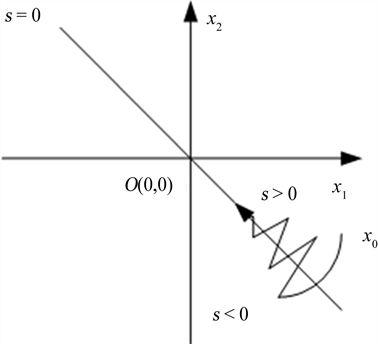Figure 1. Scheme of sliding mode control

$\left\{\begin{array}{l}{x}_{1}={v}^{*}-v\\ {x}_{2}={\int }_{-\infty }^{t}{x}_{1}\text{d}t={\int }_{-\infty }^{t}\left({v}^{*}-v\right)\text{d}t\end{array}$ (10)

$\left\{\begin{array}{l}{\stackrel{˙}{x}}_{1}=-\stackrel{˙}{v}=-\frac{{K}_{f}}{M}{i}_{q}+\frac{1}{M}f+\frac{B}{M}v\\ {\stackrel{˙}{x}}_{2}={x}_{1}={v}^{*}-v\end{array}$ (11)

$s={x}_{1}+c{x}_{2}$ (12)

$\stackrel{˙}{s}={\stackrel{˙}{x}}_{1}+c{x}_{1}=-\frac{{K}_{f}}{M}{i}_{q}+\frac{1}{M}f+\frac{B}{M}v+c{x}_{1}$ (13)

$\stackrel{˙}{s}=-\epsilon \mathrm{sgn}\left(s\right)-ks\epsilon >0,k>0$ (14)

$-\epsilon \mathrm{sgn}\left(s\right)-ks=-\frac{{K}_{f}}{M}{i}_{q}+\frac{1}{M}f+\frac{B}{M}v+c{x}_{1}$ (15)

${i}_{q}=\frac{M}{{K}_{f}}\left[c{x}_{1}+\frac{1}{M}f+\frac{B}{M}v+\epsilon \mathrm{sgn}\left(s\right)+ks\right]$ (16)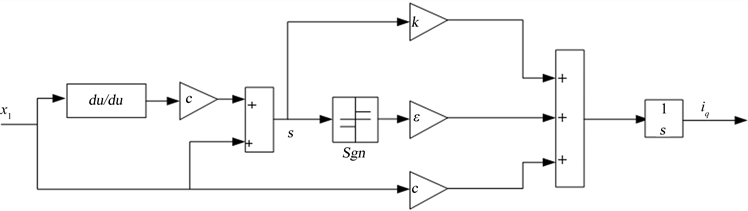Figure 2. Sliding mode controller structure diagram

3.2. 干扰观测器设计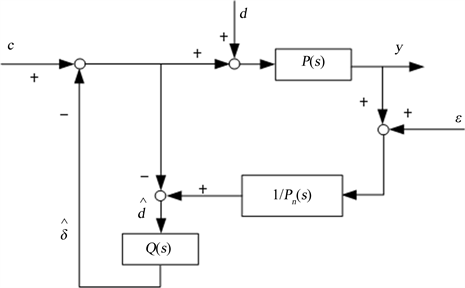Figure 3. Scheme of disturbance observer

$\stackrel{^}{\delta }=\stackrel{^}{d}=\left(1-\frac{{P}_{n}}{P}\right)c+\frac{1}{p}\xi +d$ (17)

$y=P\left(c-\stackrel{^}{d}+d\right)={P}_{n}u-\xi$ (18)

$y={G}_{c}\left(s\right)c+{G}_{d}\left(s\right)d+{G}_{\zeta }\left(s\right)\xi$ (19)

$Q\left(s\right)=\frac{3\tau s+1}{{\left(\tau s\right)}^{3}+3{\left(\tau s\right)}^{2}+3\tau s+1}$ (20)

4. 仿真与分析

4.1. 建立仿真

MATLAB是美国公司MathWorks开发的一款商业数学软件，它主要用于算法开发、数据可视化、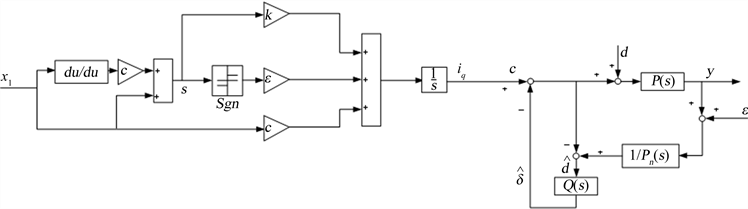Figure 4. Motion profile controllerFigure 5. Controller structure of permanent magnet synchronous linear motor

4.2. 结果分析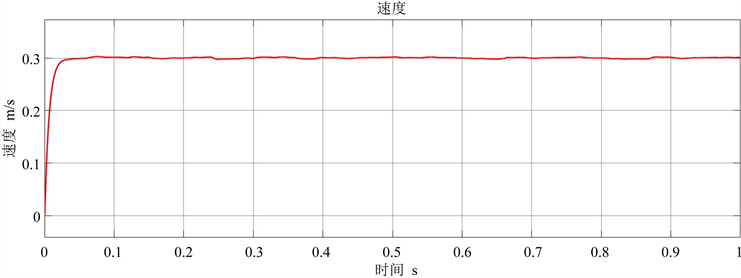(a)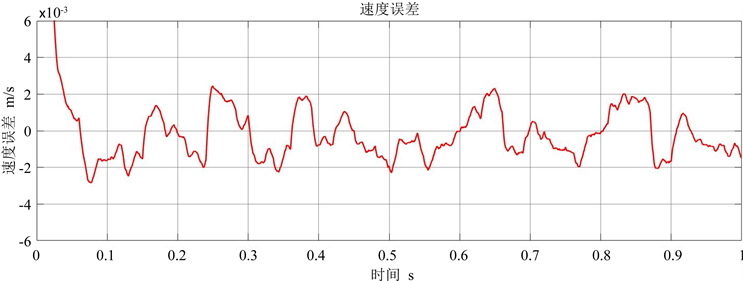(b)

Figure 6. (a) The speed of the motion platform (PID only); (b) Speed error (PID only)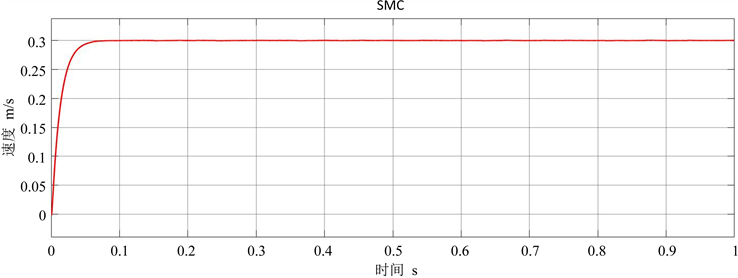(a)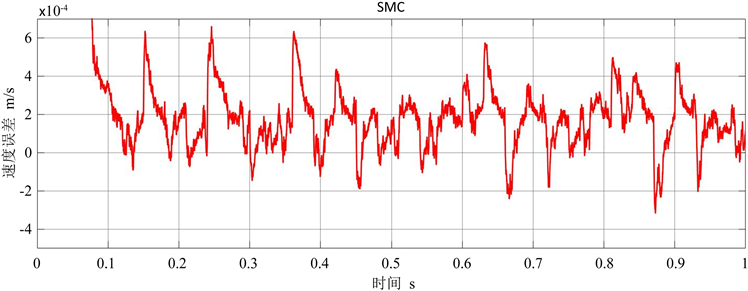(b)

Figure 7. (a) The speed of the motion platform (SMC only); (b) Speed error (SMC only)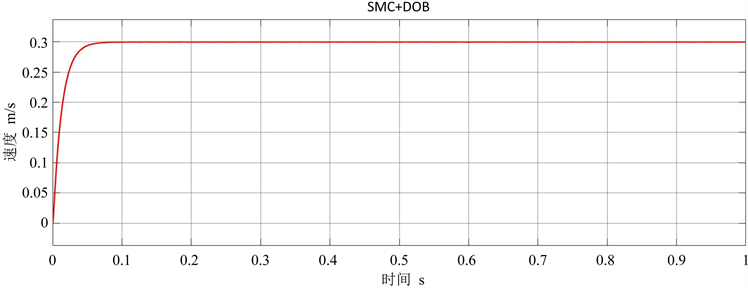(a)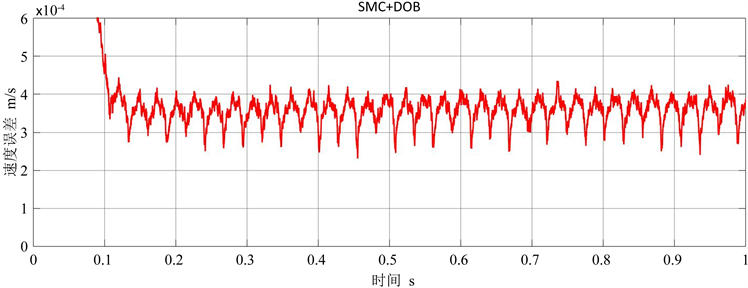(b)

Figure 8. (a) The speed of the motion platform (SMC + DOB); (b) Speed error (SMC + DOB)

5. 结论

  王先逵, 陈定积, 吴丹. 机床进给系统用直线电动机综述[J]. 制造技术与机床, 2001(8): 18-21.  赵希梅, 赵久威, 李红宜. 基于GPC和DOB的直驱XY平台鲁棒跟踪控制[J]. 电工技术学报, 2015, 30(6): 150-154.  Egiguren, P.A., Caramazana, O.B. and Etxeberria, J.A.C. (2011) Linear Generalized Predictive Position Control of Induction Motor Drives. 37th Annual Conference of the IEEE Industrial Electronics Society, Melbourne, 7-10 November 2011, 1922-1927. https://doi.org/10.1109/IECON.2011.6119600  李志军, 刘成颖, 孟凡伟, 等. 基于ZEPTC和DOB的直线电机控制器设计及实验研究[J]. 中国电机工程学报, 2012, 32(24): 134-140.  张刚, 刘品宽, 张波, 等. 直线电机精密运动平台轨迹跟踪控制器设计[J]. 光学精密工程, 2013, 21(2): 371-378.  梅雪松, 陶涛, 堤正臣, 等. 高速、高精度数控伺服工作台摩擦误差的研究[J]. 机械工程学报, 2001, 37(6): 76-81.  Lai, C.K. and Kuo, K.S. (2005) A Novel Motor Drive Design for Incremental Motion System via Sliding-Mode Control Method. IEEE Transactions on Industrial Electronics, 52, 499-507. https://doi.org/10.1109/TIE.2005.844230  罗慧, 尹泉, 孙明明. 交流伺服系统参数辨识方法综述[J]. 伺服控制, 2012(1): 27-29.  贾洪平, 贺益康. 永磁同步电机滑模变结构直接转矩控制[J]. 电工技术学报, 2006, 21(1): 1-6.  祖冉, 李敏, 黄妍慧, 何龙. 带负载观测器的永磁同步电机滑模无差拍控制[J]. 中北大学学报(自然科学版), 2022(5): 411-417.  李坤全, 邵凤翔. 一种精密运动平台轨迹跟踪控制器设计[J]. 科技通报, 2017, 33(8): 105-109.  周衡, 金超武. 基于H_∞和干扰观测器的磁悬浮轴承干扰抑制方法[J]. 机械制造与自动化, 2022, 51(3): 149-151+158.